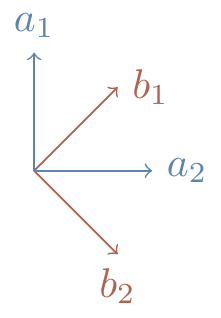## 9.4 Quantum correlations, revisited

Continuing this story of Alice and Bob with their observables and pairs of qubits, let us first rephrase things in the formalism of quantum mechanics that we’ve been building up. The observables A_1, A_2, B_1, B_2 become (2\times 2) Hermitian matrices, each with the two eigenvalues \pm 1, and \langle S\rangle becomes the expected value of the (4\times 4) CHSH matrix S = A_1\otimes(B_1-B_2) + A_2\otimes(B_1+B_2). We can now evaluate \langle S\rangle using quantum theory.

If the two qubits are in the singlet state |\psi\rangle = \frac{1}{\sqrt{2}} \left( |01\rangle-|10\rangle \right) then we have already seen (Section 9.1) that \langle A\otimes B\rangle = -\vec{a}\cdot\vec{b}. So if we choose vectors \vec{a}_1, \vec{a}_2, \vec{b}_1, and \vec{b}_2 as shown in Figure 9.1, then the corresponding matrices159 satisfy \begin{aligned} \langle A_1\otimes B_1\rangle &= \langle A_2\otimes B_1\rangle = \langle A_2\otimes B_2\rangle = \frac{1}{\sqrt{2}} \\\langle A_1\otimes B_2\rangle &= -\frac{1}{\sqrt{2}}. \end{aligned}Figure 9.1: The relative angle between the two perpendicular pairs is 45^\circ.

Plugging these values in, we get that \langle A_1 B_1\rangle - \langle A_1 B_2\rangle + \langle A_2 B_1\rangle + \langle A_2 B_2\rangle = -2\sqrt{2}, which obviously violates CHSH inequality: -2\sqrt{2} is strictly less than -2!

But here is the really important part of this discussion: this violation of the CHSH has been observed in a number of painstakingly careful experiments — this is not purely theoretical! The early efforts in these experiments were truly heroic, with many many layers of complexity; today, however, such experiments are routine.

The behaviour of entangled quantum systems cannot be explained by any local hidden variables.

1. That is, A_1=\vec{a}_1\cdot\vec{\sigma}, and so on.↩︎